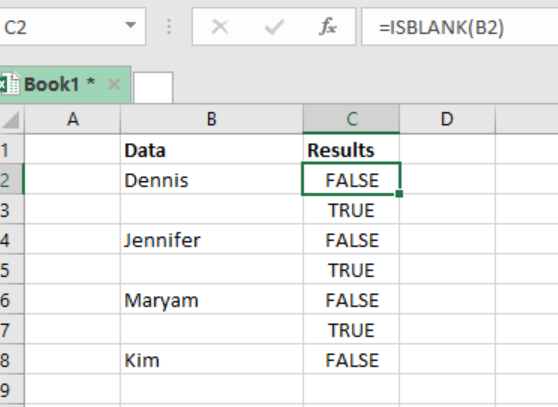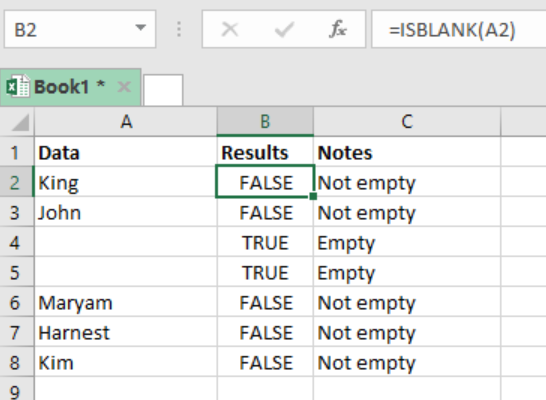Get instant live expert help with Excel or Google Sheets“My Excelchat expert helped me in less than 20 minutes, saving me what would have been 5 hours of work!”

#### Post your problem and you’ll get expert help in seconds.

Your message must be at least 40 characters
Our professional experts are available now. Your privacy is guaranteed.

# Excel ISBLANK Function

When we want to know whether cells in an excel spreadsheet are empty or not, we can use the excel ISBLANK function. This function returns TRUE if the cell is empty, and FALSE if not empty. This post provides a clear guide on how to use the Excel ISBLANK function.Figure 1: Excel ISBLANK function

## Syntax of the formula

`=ISBLANK (value)`

Where;

• Value- refers to the value we want to check. Can be a cell reference.

## Explanation of the Excel ISBLANK function

The ISBLANK function is necessary when we want to easily know those cells that are blank and those that are not in excel. We get the following results from the function;

• The function will return TRUE if the cell is empty.
• FALSE if the cell is not empty.

We can look at the ISBLANK as meaning “is empty”, as it will return a TRUE if the cell is actually empty.

Note that anything that can be in a cell, whether an empty string or a formula, the ISBLANK function will return FALSE.

## ExampleFigure 2: Examining empty and non-empty cells using ISBLANK function

Step 1: Prepare the excel sheet you want to examine.

Step 2: In cell B2, specify the formula, i.e. `=ISBLANK (A2)`. Note that A2 is a cell reference for our value.

Step 3: Press Enter to get the result.

Step 4: Copy down the formula to get result for the other cells.

## Instant Connection to an Expert through our Excelchat Service

Most of the time, the problem you will need to solve will be more complex than a simple application of a formula or function. If you want to save hours of research and frustration, try our live Excelchat service! Our Excel Experts are available 24/7 to answer any Excel question you may have. We guarantee a connection within 30 seconds and a customized solution within 20 minutes.

### Did this post not answer your question? Get a solution from connecting with the expert.Another blog reader asked this question today on Excelchat:
Solution examplesUse the Vlookup Function to complete the "employee" column of table 2. Use "job Id" from table 2 as your lookup_value(s) and table 1 as your reference.
Solved by C. H. in 16 minsIf a cell in another sheet is populated I need a vlookup done. If the cell is not populated I need the cell to return blank.
Solved by T. D. in 60 minsI am trying to make a chart that turns a week range red if nothing is entered in the range. If something is entered then I would like it to turn green. Please Help
Solved by E. U. in 43 minsI need a check box to show/hide an answer of an if function
Solved by Z. U. in 23 minsI have a cell that has a working vlook formula in it. When it looks up source data it returns blank. That is fine. I now want to use an IF formula to say if that cell is blank leave the other cell blank and if not then show value in different cell. The issue I have is that when the IF formula looks up the cell it reports back FALSE due to the vlook up formula being in the cell. I want the IF formula to show nothing instead of FALSE. Can you help?
Solved by Z. E. in 60 mins## Subscribe to Excelchat.coAnother blog reader asked this question today on Excelchat: